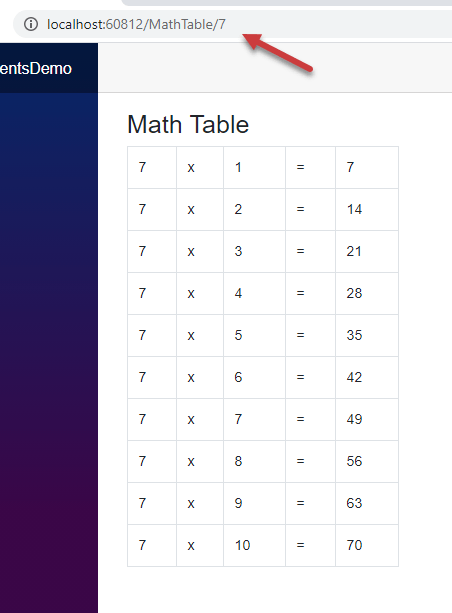Blazor 应用程序是组件的组合，这些组件不仅负责呈现用户界面，还协同工作以促进用户交互。它们是 Blazor 应用程序的主要构建块，大多数 Blazor 功能都是围绕组件展开的。在本教程中，我将向您详细介绍组件，并向您展示在 Blazor 应用程序中创建和使用组件的多种技术。

## Blazor 组件概述

Blazor 组件是 UI 的一个自包含部分，例如一个页面、一个侧边栏菜单、一个联系人表单或仪表盘小工具等。它包括用于呈现 UI 的 HTML 标签和用于处理数据或处理用户事件的 C# 代码。组件可以相互嵌套，也可以在项目中重用，甚至可以跨多个项目重用。Blazor 组件是作为 Razor 组件实现的，这正是它们使用 Razor 语法并具有 .razor 文件扩展名的原因。``````@page "/counter"

<h1>Counter</h1>

<p>Current count: @currentCount</p>

<button class="btn btn-primary" @onclick="IncrementCount">Click me</button>

@code {
private int currentCount = 0;

private void IncrementCount()
{
currentCount++;
}
}
``````

``````@page "/counter"
``````

``````@page "/counter"
@page "/mycounter"
``````

@page 指令之后，是用于指定该组件 UI 的 HTML 标记。这些标记可以使用 Razor 语法动态地使用表达式、条件或循环来渲染 HTML。在上述的 Counter 组件示例中，其 UI 包含一个标题 (h1)、一个段落 (p) 和一个按钮 (button) 元素。段落 (p) 元素使用 Razor 语法来输出 C# 代码块中定义的 currentCount 变量的值。

``````<p>Current count: @currentCount</p>
``````

``````<button class="btn btn-primary" @onclick="IncrementCount">Click me</button>
``````

``````@code {
private int currentCount = 0;

private void IncrementCount()
{
currentCount++;
}
}
``````

## 在 Visual Studio 2019 中创建 Blazor 组件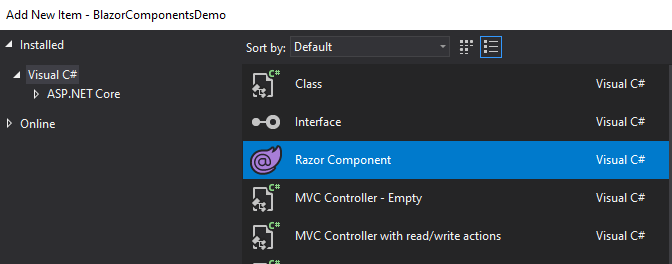Calculator.razor

``````@page "/calculator"

<h3>Calculator</h3>

<div class="form-group">
<label for="number1">Number 1</label>
<input type="number" class="form-control" id="number1" @bind="number1">
</div>
<div class="form-group">
<label for="number2">Number 2</label>
<input type="number" class="form-control" id="number2"  @bind="number2">
</div>
<div class="form-group">
<label><b>Total: </b>@total</label>
</div>

<button class="btn btn-primary" @onclick="Calculate">Calculate</button>

@code {
private int number1 = 0;
private int number2 = 0;
private int total = 0;

private void Calculate()
{
total = number1 + number2;
}
}
``````

@code 块具有三个私有变量和一个 Calculate 方法。Calculate 方法简单地将 number1number2 的和赋值给 total 变量。

HTML 标记中有两个输入框，它们使用 @bind 属性来绑定 number1number2 变量：

``````<input type="number" class="form-control" id="number1" @bind="number1">
``````

``````<label><b>Total: </b>@total</label>
``````

``````<button class="btn btn-primary" @onclick="Calculate">Calculate</button>
``````

``````<li class="nav-item px-3">
<span class="oi oi-calculator" aria-hidden="true"></span> Calculator
</li>
``````

`F5` 运行您的应用程序，您应该会看到如下所示的页面。尝试在输入框中输入一些数字，您应该能看到页面上显示的数字之和。点击 Calculate 按钮运行了服务端的 C# 代码，却并没有浏览器回传或页面刷新。一切都感觉那么流畅和快速，就像您在浏览器中使用 JavaScript 进行计算一样。## 拆分 Blazor 组件中的标签和代码

### 使用基类拆分组件

CalculatorBase.cs

``````public class CalculatorBase : ComponentBase
{
private int number1 = 0;
private int number2 = 0;
private int total = 0;

private void Calculate()
{
total = number1 + number2;
}
}
``````

Calculator.razor

``````@page "/calculator"
@inherits CalculatorBase

<h3>Calculator</h3>

<div class="form-group">
<label for="number1">Number 1</label>
<input type="number" class="form-control" id="number1" @bind="number1">
</div>
<div class="form-group">
<label for="number2">Number 2</label>
<input type="number" class="form-control" id="number2"  @bind="number2">
</div>
<div class="form-group">
<label><b>Total: </b>@total</label>
</div>

<button class="btn btn-primary" @onclick="Calculate">Calculate</button>

@code {

}
``````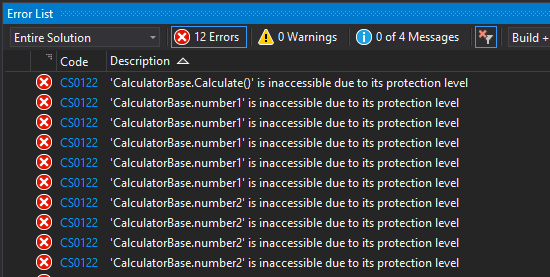``````public class CalculatorBase : ComponentBase
{
public int number1 = 0;
public int number2 = 0;
public int total = 0;

public void Calculate()
{
total = number1 + number2;
}
}
``````

💡 基类中的字段和方法改为 `protected`， 在 `.razor` 中也是可以的访问的。
💡 CalculatorBase 类必须包含在一个命名空间中，否则会报错。

### 使用部分类拆分组件

Blazor 组件生成为部分类，这意味着我们可以创建一个与我们的组件同名的 partial 类，并将所有 C# 代码移动到该部分类中。然后，此部分类将成为代码隐藏文件，该文件中声明的字段和属性可在 Blazor 组件中直接使用。让我们创建一个类 Calculator.razor.cs，并将 Calculator 的代码放在这个新类中。

Calculator.razor.cs

``````public partial class Calculator
{
public int number1 = 0;
public int number2 = 0;
public int total = 0;

public void Calculate()
{
total = number1 + number2;
}
}
``````## 创建和使用子组件

Blazor 子组件是没有 @page 指令的组件。这些组件可以使用标准的 HTML 语法包含在其他组件中。然后，我们可以通过在页面上添加组件来构建复杂的 UI，我们甚至可以在同一个页面上添加同一子组件的多个实例。如果一个子组件可能在多个父组件或页面中重复使用，那么最好将它放在 Shared 文件夹中。让我们在 Shared 文件夹中创建一个简单的 Heading.razor 子组件，并在其中添加以下代码。

``````<h3>Calculator</h3>
``````

Calculator.razor

``````@page "/calculator"
@inherits CalculatorBase

<div class="form-group">
<label for="number1">Number 1</label>
<input type="number" class="form-control" id="number1" @bind="number1">
</div>
<div class="form-group">
<label for="number2">Number 2</label>
<input type="number" class="form-control" id="number2"  @bind="number2">
</div>
<div class="form-group">
<label><b>Total: </b>@total</label>
</div>

<button class="btn btn-primary" @onclick="Calculate">Calculate</button>
``````

``````<Heading />
``````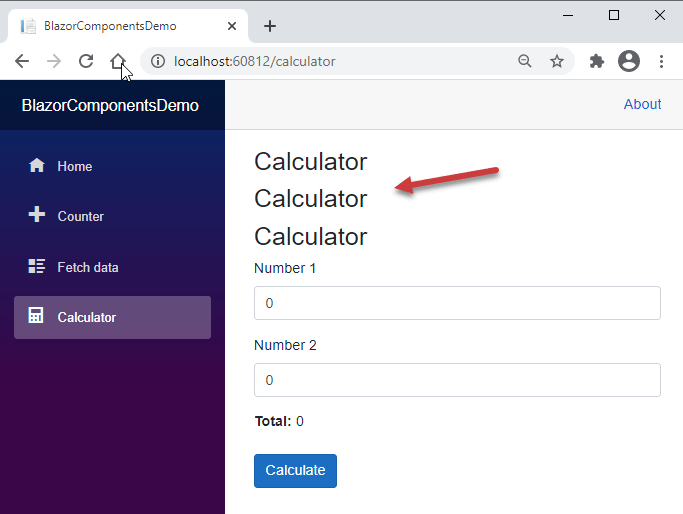## 自定义带参数的 Blazor 组件

``````<h3>@Title</h3>

@code {
[Parameter]
public string Title { get; set; } = "Default Title";
}
``````Visual Studio 智能感知也可以提示组件参数，因此我们不需要记忆这些参数，这很有用。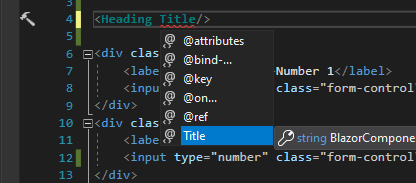``````<Heading Title="Calculator" />
``````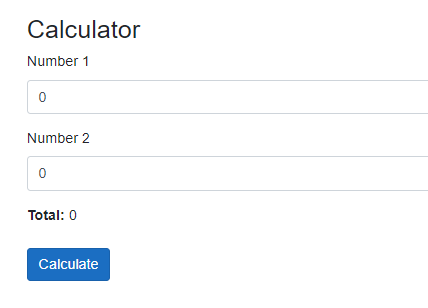CalculatorTotal.razor

``````<label><b>Total: </b>@Total</label>

@code {
[Parameter]
public int Total { get; set; }
}
``````

Calculator.razor

``````@page "/calculator"

<div class="form-group">
<label for="number1">Number 1</label>
<input type="number" class="form-control" id="number1" @bind="number1">
</div>
<div class="form-group">
<label for="number2">Number 2</label>
<input type="number" class="form-control" id="number2" @bind="number2">
</div>
<div class="form-group">
<CalculatorTotal Total="@total"/>
</div>

<button class="btn btn-primary" @onclick="Calculate">Calculate</button>
``````

## 将路由参数传递给 Blazor 组件

Blazor 组件还可以接受在 @page 指令中提供的来自路由模板的参数。路由器使用路由参数自动填充相应的组件参数。

MathTable.razor

``````@page "/MathTable/{number:int}"

<h3>Math Table</h3>

<table class="table table-bordered w-25">
@for (int i = 1; i <= 10; i++)
{
<tr>
<td>@Number</td>
<td>x</td>
<td>@i</td>
<td>=</td>
<td>@(Number * i)</td>
</tr>
}
</table>

@code {
[Parameter]
public int Number { get; set; }
}
``````

``````@page "/MathTable/{number:int}"
``````

``````[Parameter]
public int Number { get; set; }
``````